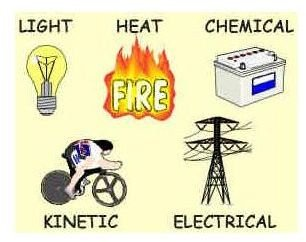# Forms of Energy Include Kinetic Energy, Potential Energy, and Thermal Energy

Energy is commonly defined as the capacity of a system or a body to do work. There are many forms of energy (to be described later), but any of them can be used to do work. For example the chemical energy in gasoline can be burned in an automobile engine to make the car move. Electrical energy can be used to turn a motor and make machinery move. The thermal energy in the steam used in an electric power plant can partially be converted to mechanical energy in a turbine and then converted to electrical energy in a generator.

## Common Forms of EnergyThere are two possible forms of energy in physics, kinetic energy and potential energy. In common usage, however, this is expanded to many possible forms of energy. Some of the more common forms are described here.

Kinetic Energy is the energy that any moving object has by virtue of its motion. The kinetic energy of a moving object is one half of its mass times the square of its velocity (K.E. = (1/2)mV2).

Image Credit: Alistair Craven

Potential Energy is stored energy that has the potential to do work or be converted to another form. An object that can move downward under the force of gravity has gravitational potential energy. Also, a compressed spring and a stretched rubber band have potential energy, because they can do work as they move back to their natural position.

Thermal Energy, commonly simply called heat, is the energy of any object with a temperature above absolute zero, due to the kinetic energy of its molecules. For a given quantity of material, the higher its temperature, the more thermal energy it has.

Chemical Energy is a particular form of potential energy. It is the energy stored in a chemical that can be released by some reaction of that chemical. An example is the chemical energy in coal that is converted to thermal energy when the coal is oxidized (burned).

Electrical Energy is energy that is made available due to the flow of an electrical current through a conductor. Electrical energy is actually energy in transit. For example, it is converted to heat when electrical current passes through a resistor, or it is converted to kinetic energy when electrical current passes through the windings of an electrical motor, causing the motor to turn.

Mechanical Energy is a term used to refer to the sum of the kinetic energy and potential energy of a system.

## Energy Conservation and Conversion among the Forms of Energy

The first law of thermodynamics is an expression of the conservation of energy. It states that energy can be neither created nor destroyed (in the absence of nuclear reactions). Energy can, however, be converted from one form to another and can be used to do work. Some examples of energy conversion are as follows.

Potential energy to kinetic energy: If a car at rest at the top of a hill is allowed to coast down the hill, most its initial potential energy will have been converted to the kinetic energy of the moving car at the bottom of the hill. Some of the initial potential energy will have been converted to thermal energy (heat) due to friction between moving parts.

Chemical energy to electrical energy: A battery contains stored chemical energy that can be converted to electrical energy by connecting an electrical conductor across the terminals of the battery. The electrical energy will in turn be converted to heat or mechanical energy, depending upon what kind of device is connected across the terminals of the battery.

Chemical energy to thermal energy: When natural gas is burned in a furnace the chemical energy in the fuel is converted to heat (thermal energy) to keep a building warm.

I'm sure you can think of many more examples of energy conversion from one form to another.

## Non-Renewable and Renewable Energy Sources

In relatively recent times, the classification of energy sources as either nonrenewable or renewable energy sources has become increasingly important. The huge quantity of the fossil fuels (coal, petroleum, and natural gas) stored underground seemed to provide an infinite supply of readily usable energy when they were first discovered and put to use. As the rate of use of fossil fuels has mushroomed, however, we've come to realize that there is a finite supply that is diminishing. As a result, the importance of developing and using renewable energy resources, like solar energy, wind energy, and biomass energy has become more prominent.

## References

2. University of Wisconsin – Stevens Point, College of Natural Resources website

3. Nixon, James (editor), Forms of Energy, Chicago, IL, Raintree. 2010